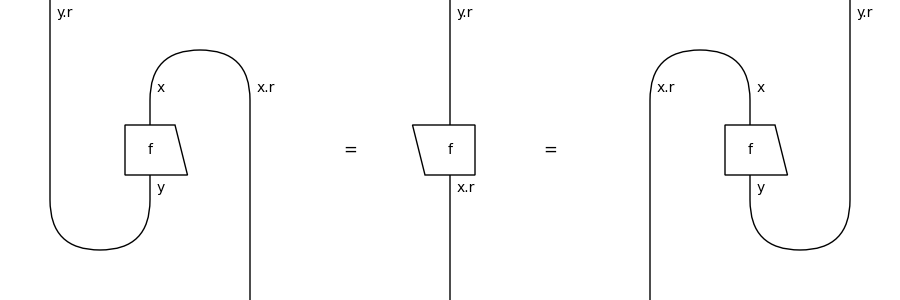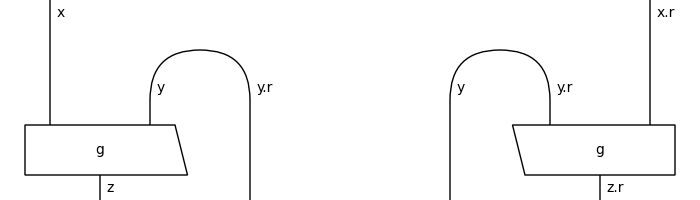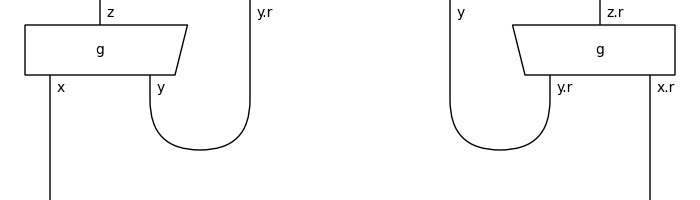# pivotal#

The free pivotal category, i.e. diagrams with cups and caps that can rotate by a full turn.

## Summary#

 `Ob` A pivotal object is a rigid object where left and right adjoints coincide. `Ty` A pivotal type is a rigid type with pivotal objects inside. `Diagram` A pivotal diagram is a rigid diagram and a traced diagram with pivotal types as domain and codomain. `Box` A pivotal box is a rigid box in a pivotal diagram. `Cup` A pivotal cup is a rigid cup of pivotal types. `Cap` A pivotal cap is a rigid cap of pivotal types. `Category` A pivotal category is a rigid category where left and right adjoints coincide. `Functor` A pivotal functor is a rigid functor on a pivotal category.

## Axioms#

A pivotal category is a rigid category where left and right transpose coincide.

```>>> x, y, z = map(Ty, "xyz")
>>> assert x.r == x.l and x.l.l == x == x.r.r
>>> f = Box('f', x, y)
```
```>>> from discopy.drawing import Equation
>>> Equation(f.transpose(left=True), f.r, f.transpose(left=False)).draw(
...     figsize=(6, 3), path="docs/_static/pivotal/axiom.png")
```For each diagram, we have its conjugate:

```>>> d = Box('g', x @ y, z).curry()
>>> Equation(d, d.conjugate(), symbol="").draw(
...     figsize=(6, 2), space=2, path="docs/_static/pivotal/box-conjugate.png")
```We also have its dagger and its transpose:

```>>> Equation(d.dagger(), d.rotate(), symbol="").draw(
...     figsize=(6, 2), space=2,
...     path="docs/_static/pivotal/dagger-transpose.png")
```